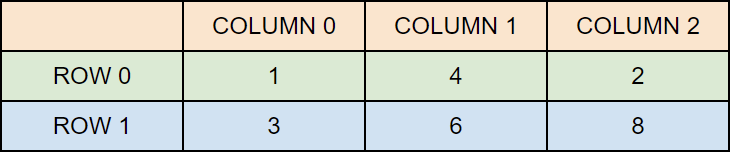# C# Multidimensional Arrays

## Multidimensional Arrays

In the previous chapter, you learned about arrays, which is also known as single dimension arrays. These are great, and something you will use a lot while programming in C#. However, if you want to store data as a tabular form, like a table with rows and columns, you need to get familiar with multidimensional arrays.

A multidimensional array is basically an array of arrays.

Arrays can have any number of dimensions. The most common are two-dimensional arrays (2D).

## Two-Dimensional Arrays

To create a 2D array, add each array within its own set of curly braces, and insert a comma (`,`) inside the square brackets:

### Example

``int[,] numbers = { {1, 4, 2}, {3, 6, 8} };``

Good to know: The single comma `[,]` specifies that the array is two-dimensional. A three-dimensional array would have two commas: `int[,,]`.

`numbers` is now an array with two arrays as its elements. The first array element contains three elements: 1, 4 and 2, while the second array element contains 3, 6 and 8. To visualize it, think of the array as a table with rows and columns:## Access Elements of a 2D Array

To access an element of a two-dimensional array, you must specify two indexes: one for the array, and one for the element inside that array. Or better yet, with the table visualization in mind; one for the row and one for the column (see example below).

This statement accesses the value of the element in the first row (0) and third column (2) of the `numbers` array:

### Example

``````int[,] numbers = { {1, 4, 2}, {3, 6, 8} };
Console.WriteLine(numbers[0, 2]);  // Outputs 2``````

Remember that: Array indexes start with 0:  is the first element.  is the second element, etc.

## Change Elements of a 2D Array

You can also change the value of an element.

The following example will change the value of the element in the first row (0) and first column (0):

### Example

``````int[,] numbers = { {1, 4, 2}, {3, 6, 8} };
numbers[0, 0] = 5;  // Change value to 5
Console.WriteLine(numbers[0, 0]); // Outputs 5 instead of 1``````

## Loop Through a 2D Array

You can easily loop through the elements of a two-dimensional array with a `foreach` loop:

### Example

``````int[,] numbers = { {1, 4, 2}, {3, 6, 8} };

foreach (int i in numbers)
{
Console.WriteLine(i);
} ``````

You can also use a for loop. For multidimensional arrays, you need one loop for each of the array's dimensions.

Also note that we have to use `GetLength()` instead of `Length` to specify how many times the loop should run:

### Example

``````int[,] numbers = { {1, 4, 2}, {3, 6, 8} };

for (int i = 0; i < numbers.GetLength(0); i++)
{
for (int j = 0; j < numbers.GetLength(1); j++)
{
Console.WriteLine(numbers[i, j]);
}
}  ``````

W3Schools is optimized for learning and training. Examples might be simplified to improve reading and learning. Tutorials, references, and examples are constantly reviewed to avoid errors, but we cannot warrant full correctness of all content. While using W3Schools, you agree to have read and accepted our terms of use, cookie and privacy policy.